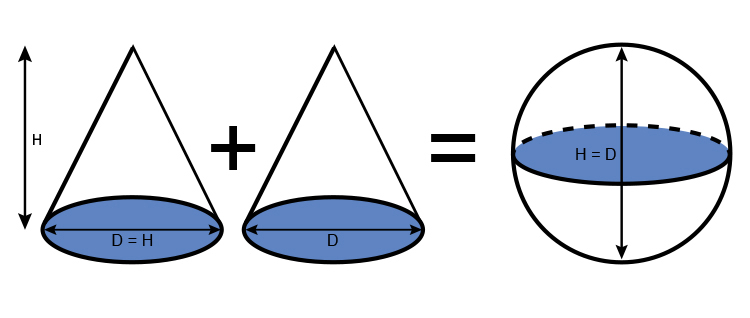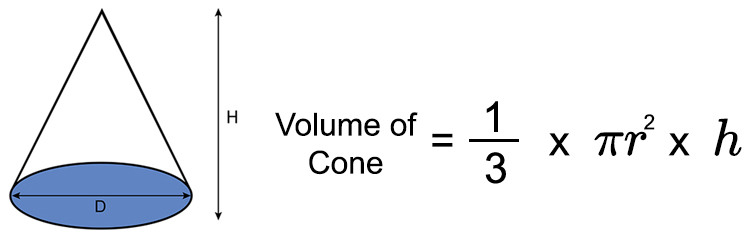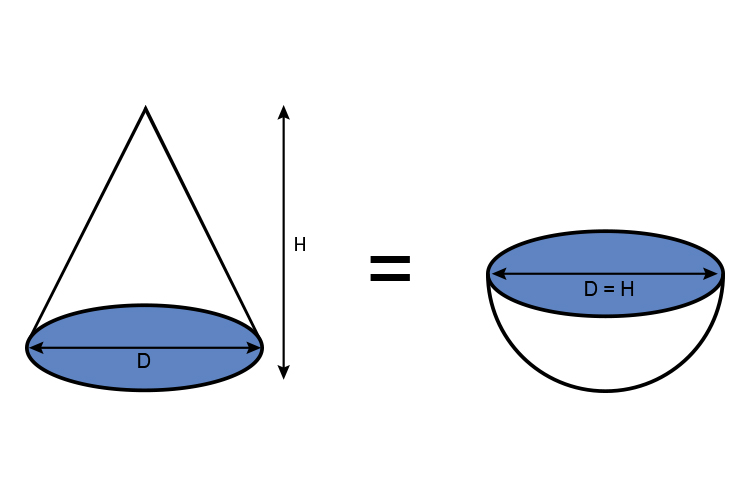# Volume of a sphere

The volume of a sphere is 4/3\pir^3

You may look at this and ask how am I going to remember this formula but actually, it’s quite easy to work out, it takes seconds: Just remember: Two cones = sphere:NOTE:

The areas shaded are all the same size (that is D=H).

We know that volume of a cone isVolume of cone = 1/3\timesπr^2\timesh

Therefore volume of a sphere:

(1/3\times\pir^2\timesh)+(1/3\times\pir^2\timesh)= Volume of a sphere

As h = 2r then

(1/3\times\pir^2\times2r)+(1/3\times\pir^2\times2r)= Volume of a sphere

And that’s the same as:

(2/3\times\pir^3)+(2/3\times\pir^3)= Volume of a sphere

Which again is the same as:

2(2/3\times\pir^3)= Volume of a sphere

(2\times2)/3\timespir^3= Volume of a sphere

And

4/3\timespir^3 = Volume of a sphere

NOTE:

The volume of one cone must be the same volume as a hemisphere (if they have the same radius and the height of the cone is the diameter of the sphere).

Hemisphere = 1/2 a sphere